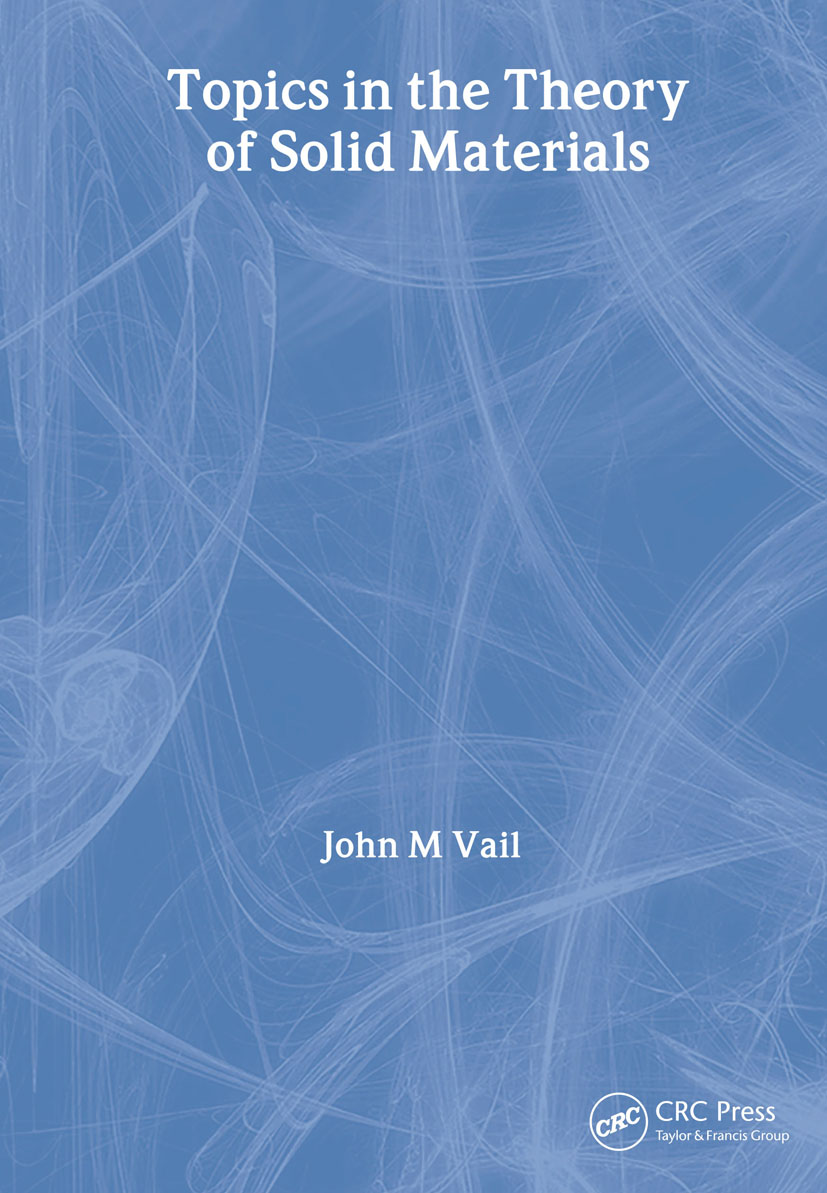# Topics in the Theory of Solid Materials

## 1st Edition

CRC Press

368 pages

##### Purchasing Options:\$ = USD
Paperback: 9780750307291
pub: 2003-04-24
Currently out of stock
\$85.95
x
eBook (VitalSource) : 9781315274614
pub: 2018-10-08
from \$39.98

FREE Standard Shipping!

### Description

Topics in the Theory of Solid Materials provides a clear and rigorous introduction to a wide selection of topics in solid materials, overlapping traditional courses in both condensed matter physics and materials science and engineering. It introduces both the continuum properties of matter, traditionally the realm of materials science courses, and the quantum mechanical properties that are usually more emphasized in solid state physics courses, and integrates them in a manner that will be of use to students of either subject. The book spans a range of basic and more advanced topics, including stress and strain, wave propagation, thermal properties, surface waves, polarons, phonons, point defects, magnetism, and charge density waves.

Topics in the Theory of Solid Materials is eminently suitable for graduates and final-year undergraduates in physics, materials science, and engineering, as well as more advanced researchers in academia and industry studying solid materials.

### Reviews

"What are dislocations? What are phonons? What is phonon transport? This text describes all that and more in lucid language. If you're into materials and would like to relearn the undergraduate condensed matter physics that you wished you knew, this is the book to read."

-Biswajit Banerjee, University of Utah, Salt Lake City, USA

STRAIN AND STRESS IN CONTINUOUS MEDIA

Introduction

Deformation: Strain and Rotation

Forces and Stress

Linear Elasticity

Equilibrium

WAVE PROPAGATION IN CONTINUOUS MEDIA

Introduction

Vector Fields

Equation of Motion

Wave Propagation

Appendix

THERMAL PROPERTIES OF CONTINUOUS MEDIA

Introduction

Classical Thermodynamics

Thermal Conduction and Wave Motion

Wave Attenuation by Thermal Conduction

SURFACE WAVES

Introduction

Rayleigh Waves

Boundary Conditions

Dispersion Relation

Character of the Wave Motion

DISLOCATIONS

Introduction

Description of Dislocations

Deformation Fields of Dislocations

Uniform Dislocation Motion

Further Study of Dislocations

CLASSICAL THEORY OF THE POLARON

Introduction

Equations of Motion

The Constant-Velocity Polaron

Polaron in a Magnetic Field: Quantization

ATOMISTIC QUANTUM THEORY OF SOLIDS

Introduction

The Hamiltonian

Nuclear Dynamics: The Adiabatic Approximation

The Harmonic Approximation

Phonons

Statistical Thermodynamics of a Solid

Summary

PHONONS

Introduction

Monatomic Linear Chain

Diatomic Linear Chain

Localized Mode of a Point Defect

CLASSICAL ATOMISTIC MODELING OF CRYSTALS

Introduction

The Shell Model for Insulating Crystals

Cohesive Energy of a Crystal

Elastic Constants

Dielectric and Piezoelectric Constants

CLASSICAL ATOMIC DIFFUSION IN SOLIDS

Introduction

The Diffusion Equation

Diffusion as a Random Walk

Equilibrium Distribution of Point Defects

Temperature Dependence of Diffusion: the Vineyard Relation

Appendix: Stirling's Formula

POINT DEFECTS IN CRYSTALS

Introduction

Classical Diffusion

Defect Complex Stability

Impurity Charge-State Stability

Optical Excitation

Spin Densities

Local Band-Edge Modification

Electronic Localization

Quantum Diffusion

Effective Force Constants for Local Modes

Summary

Appendix: The ICECAP Method

THEORETICAL FOUNDATIONS OF MOLECULAR CLUSTER COMPUTATIONS

Introduction

Hartree-Fock Approximation

The Fock Equation

Localizing Potentials

Embedding in a Crystal

Correlation

One-, Two-, and N-Particle Density Functionals

PARAMAGNETISM AND DIAMAGNETISM IN THE ELECTRON GAS

Introduction

Paramagnetism of the Electron Gas

Diamagnetism of the Electron Gas

Appendix

CHARGE DENSITY WAVES IN SOLIDS

Introduction

Effective Electron-Electron Interaction

The Hartree Equation: Uniform and Periodic Cases

Charge Density Waves: the Mathieu Equation

Discussion

References

### Subject Categories

##### BISAC Subject Codes/Headings:
SCI077000
SCIENCE / Solid State Physics
TEC021000
TECHNOLOGY & ENGINEERING / Material Science